# Index Scan

This method of selection uses indexes to traverse the record. The search key should be indexed and should be used in the filter condition for an Index scan to work. We have different types of index scan based on primary key and the type of operator used in the condition.

## Primary Key index with equality

In this method search key value will be the primary key and index will be created on it. In the WHERE clause we will have equality comparison with the primary key. For example, SELECT * FROM STUDENT WHERE STD_ID = 105;

These types of indexes are usually stored in B+ tree structure with height ‘h’. Then the worst case cost of the query is given as (h+1)* (ts+ tT). Here (h+1) is given because h seek to traverse the B+ tree of height h and we need one seek to traverse the data file where the record is stored.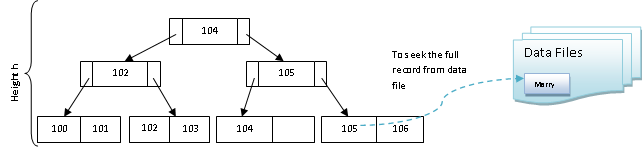## Primary key index with comparison

In this method comparison operators like <, <=, >, and >= are used to retrieved more than one record from the file. This comparison operator is used on the primary key, on which index is also created.

For example, SELECT * FROM STUDENT WHERE STD_ID >= 105; Since the index is on primary key, records are already in sorted based on the key. So, the parser has to seek the first record that is satisfying STD_ID = 105. Then it has to add the number of blocks which satisfies >= conditions.

Hence in this type of selection, one seek time and the B number of blocks before (< or <=) or after (> or >=) should be added. Usually any condition with < or <= will not use index, instead they will be sequentially searched. Only > or >= will use index to locate the first record and thereafter sequential search is done on blocks.

STD_ID>=105 will have seek time to locate 105 in the tree below and transform time for the same. Then it searches 106 sequentially and it will involve only transform time for 106 will be involved. Hence the cost is (h+1)* (ts+ tT) + n* tT, where n is the number of records after >= condition.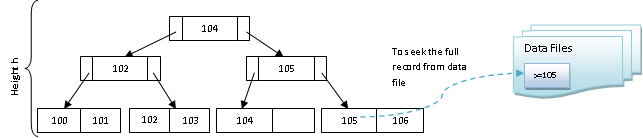## Non-Primary key Index with equality

This is similar to Primary Key index with equality, but here the index is not on any key columns. Hence it can have more than one match and return multiple records.  Hence we will have B blocks of records to return. Hence the cost of query becomes h* (ts+ tT) + B* (ts+ tT).

That is, seek and traversal time for B+ tree of height h to get the index of the record. Then we have to seek B times the data files and traverse B times all the fetched records from the data files.

For example, SELECT * FROM STUDENT WHERE STD_NAME = ‘Marry’; There will be any number of students with name Marry. They will have different STD_ID, but same name. Hence it retrieves multiple records stored in different set of memory blocks.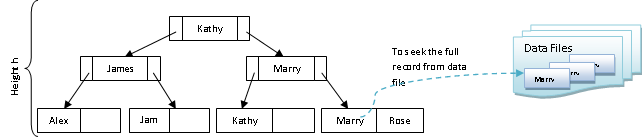Secondary Index with equality: – Here the index is created on the attribute which is neither a primary key nor a search key column.

For example, SELECT * FROM STUDENT WHERE ADDRESS = ‘Troy; where primary key is STD_ID, index is on STD_NAME and search key is ADDRESS. Here it will retrieve multiple records. Each record will be in different blocks, say n, in the memory. Hence the cost of query would be (h + n)* (ts+ tT), which is very expensive cost.

This method is different from Primary key index with comparison. Here, it is with equality operator and it has to seek one record from the tree of height h. But it has to transfer n blocks of records from the data file.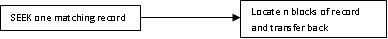Where as in Primary key index with comparison, it has to seek the first record from the tree of height h, and transfer it back. At the same time it has to search rest of the records sequentially and transfer it back from n block from the memory.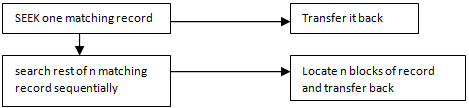If the search key is the primary key / candidate key (note that index is created on some other column), then the cost would be (h + 1)* (ts+ tT), where n=1 since we will have only one block of matching record.

## Secondary Index with comparison

This is same as secondary Index with equality but with comparison operator.
For example, SELECT * FROM STUDENT WHERE AGE>=18; where STD_ID is the primary key, STD_NAME is the index column and AGE is the comparison column / search key column. Here the comparison works similar to primary key index with comparison. One seek is required to locate AGE = 18. And rest of the matching records is searched sequentially. If the operator is < or <= then sequential search from the beginning of file till it reaches > or >= will be performed. Since the index is not on the search key column, there will be disk I/O for each of the record.

The cost would be (h+ n)* (ts+ tT) + m* tT, where n is the number of blocks of records matching first record i.e.; AGE = 18 and m is the number of blocks of records searched sequentially i.e.; AGE>18.

Translate »